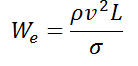# Weber number Calculator

This CalcTown calculator calculates Weber Number, which is used to analyse fluid flows when there is an interface between two different fluids

Kg/m3
m/s
m
N/m

#### Resultwhere

We:Weber Number, dimensionless
ρ: Fluid Density, in Kg/m^3
v: Fluid Speed (velocity), in meter/second
L: Characteristic Length, in meter
σ: Surface Tension, in N/m